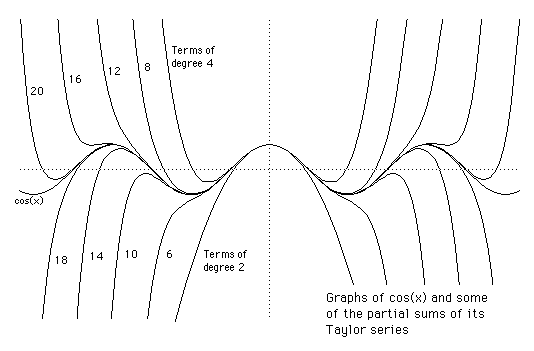# Taylor Series

Many functions can be written as powers series about some point of their domain. About a point $a$ (say) we get
$f(a + h) = f(a) + \large\frac{1}{1!}\normalsize f'(a)h + \large\frac{1}{2!}\normalsize f''(a)h^{2} + \large\frac{1}{3!}\normalsize f'''(a)h^{3} + ...$
which we call the Taylor series of $f$.

The case $a=0$ is known as the Maclaurin series of $f$.

The Taylor Series for $\sin(x)$ and $\cos(x)$ are shown below.